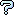All about flooble | fun stuff | Get a free chatterbox | Free JavaScript | Avatarsperplexus dot infoSemi-Minimalist Painting (Posted on 2006-03-13)A semi-minimalist painter created a work which consisted of a 6 x 6 array of small colored squares. Each small square contained just one color.

At the art gallery, six girl students were examining the painting. Each girl chose to report on exactly one horizontal row of small squares, by assigning a different number to each color in that row. The six row patterns, in the original order, were

```121341
112213
123221
121222
122113
122134
```
The girls did not consult one another, so a given digit in one row does not necessarily represent the same color as the same digit in a different row.

Another group of six girls did the same process, but this time for the columns, rather than the rows. The column patterns they came up with look like this (but the array below shows the columns in no particular order):

```1  1  1  1  1  1
2  1  2  2  1  2
3  2  3  2  2  2
4  3  2  2  3  1
3  2  1  2  4  2
2  4  1  3  3  2
```
Remember: the rows in the first table are shown in the correct order, but the columns in the second table are shown randomly. Outside of the particular row, for the first table, or column for the second table, do not expect the same digit-to-color coding scheme.

There were more green squares than any other color. How many squares were painted green?

 Submitted by Charlie Rating: 3.2000 (5 votes) Solution: (Hide) The pattern of colors, using letters this time and the same coding scheme for the whole table, is: ```abacda bbaabc ca aac bcbccc a aac accab ``` The blank spaces could be either d or a fifth color, e. The sequence of columns from the original table of columns is 352614. There are 13 a's, 7 b's, 11 c's, 1 d and 4 blanks. Even if the blanks are all d's, there are more a's than any other letter, and so a represents green and there were 13 green squares on the painting. ```DECLARE SUB permute (a\$) DATA 1,2,1,3,4,1 DATA 1,1,2,2,1,3 DATA 1,2,3,2,2,1 DATA 1,2,1,2,2,2 DATA 1,2,2,1,1,3 DATA 1,2,2,1,3,4 DATA 1,2,3,4,3,2 DATA 1,1,2,3,2,4 DATA 1,2,3,2,1,1 DATA 1,2,2,2,2,3 DATA 1,1,2,3,4,3 DATA 1,2,2,1,2,2 DIM SHARED colList\$ DIM SHARED byRow(6, 6) DIM SHARED byCol(6, 6) DIM SHARED currRow, currCol colList\$ = "123456" FOR r = 1 TO 6 FOR c = 1 TO 6 READ byRow(r, c) NEXT NEXT FOR c = 1 TO 6 FOR r = 1 TO 6 READ byCol(r, c) NEXT NEXT rc = 1: cc = 1 h\$ = colList\$ DO currRow = 1: currCol = 1: nextLet = 1 REDIM SHARED board\$(6, 6) FOR c = 1 TO 6 board\$(1, c) = MID\$("abacda", c, 1) NEXT good = 1 didSome = 1 DO didSome2 = didSome didSome = 0 FOR c = 1 TO 6 cType = VAL(MID\$(colList\$, c, 1)) FOR r = 1 TO 6 IF board\$(r, c) > "" THEN FOR r2 = 1 TO 6 IF byCol(r2, cType) = byCol(r, cType) AND r2 <> r THEN IF board\$(r2, c) > "" AND board\$(r2, c) <> board\$(r, c) THEN good = 0: EXIT FOR END IF IF board\$(r2, c) = "" THEN didSome = 1 board\$(r2, c) = board\$(r, c) END IF IF byCol(r2, cType) <> byCol(r, cType) AND r2 <> r THEN IF board\$(r2, c) > "" AND board\$(r2, c) = board\$(r, c) THEN good = 0: EXIT FOR END IF END IF NEXT IF good = 0 THEN EXIT FOR END IF NEXT IF good = 0 THEN EXIT FOR NEXT IF good = 0 THEN EXIT DO FOR r = 1 TO 6 FOR c = 1 TO 6 IF board\$(r, c) > "" THEN FOR c2 = 1 TO 6 IF byRow(r, c2) = byRow(r, c) AND c2 <> c THEN IF board\$(r, c2) > "" AND board\$(r, c2) <> board\$(r, c) THEN good = 0: EXIT FOR END IF IF board\$(r, c2) = "" THEN didSome = 1 board\$(r, c2) = board\$(r, c) END IF IF byRow(r, c2) <> byRow(r, c) AND c2 <> c THEN IF board\$(r, c2) > "" AND board\$(r, c2) = board\$(r, c) THEN good = 0: EXIT FOR END IF END IF NEXT IF good = 0 THEN EXIT FOR END IF NEXT IF good = 0 THEN EXIT FOR NEXT IF good = 0 THEN EXIT DO LOOP UNTIL didSome2 = 0 IF good THEN REDIM lct(5) FOR r = 1 TO 6 FOR c = 1 TO 6 board\$(r, c) = LEFT\$(board\$(r, c) + " ", 1) PRINT board\$(r, c); s = INSTR(" abcd", board\$(r, c)) - 1 lct(s) = lct(s) + 1 NEXT PRINT NEXT ct = ct + 1 FOR i = 0 TO 4: PRINT lct(i); : NEXT PRINT "------"; ct; colList\$ END IF permute colList\$ LOOP UNTIL colList\$ = h\$ ``` The permute subroutine is shown elsewhere on this site. The puzzle is a rewording of Enigma 1375: Patterns of Colours; New Scientist, 21 January 2006.Subject Author Dateplease explain solution process Cynthia 2006-06-29 00:47:36re: Where is this from? Charlie 2006-03-17 07:56:25Where is this from? Tristan 2006-03-14 20:46:01re(3): Solution tomarken 2006-03-14 14:59:17re(2): Solution tomarken 2006-03-14 14:45:52re: Solution Magda 2006-03-14 14:16:27re: Solution tomarken 2006-03-14 12:01:23re: Solution Soumitra Pal 2006-03-14 11:58:14Solution Soumitra Pal 2006-03-14 11:53:58Solution tomarken 2006-03-13 16:14:45Please log in:

 Search: Search body:
Forums (0)